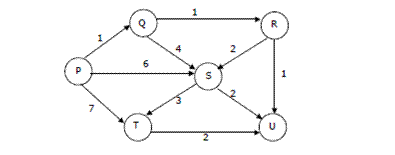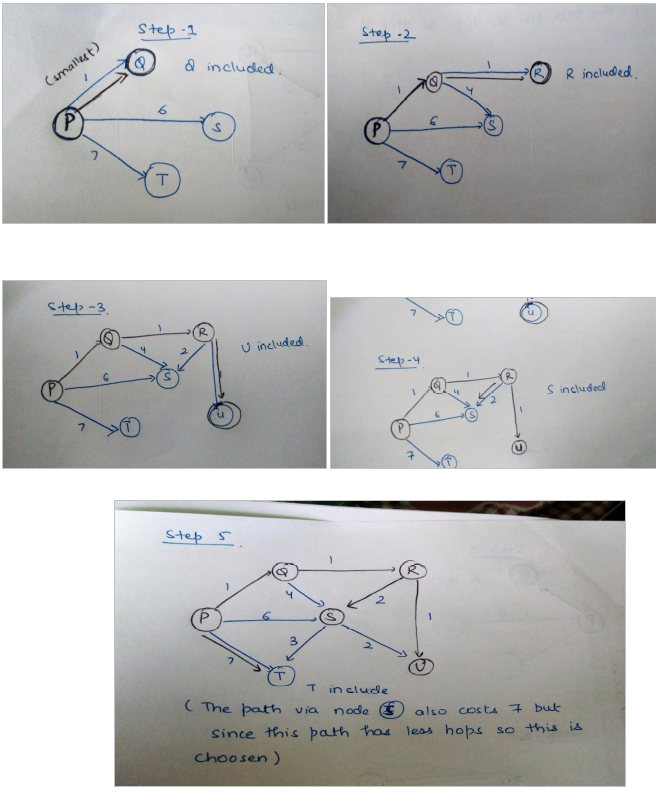Open In App

# GATE | GATE-CS-2004 | Question 44

Suppose we run Dijkstra’s single source shortest-path algorithm on the following edge weighted directed graph with vertex P as the source. In what order do the nodes get included into the set of vertices for which the shortest path distances are finalized?(A) P, Q, R, S, T, U
(B) P, Q, R, U, S, T
(C) P, Q, R, U, T, S
(D) P, Q, T, R, U, S